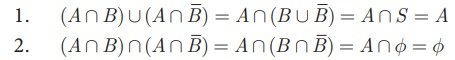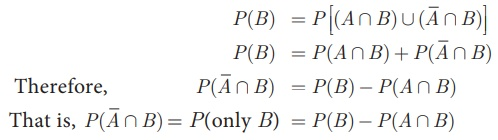Home | | Maths 10th Std | Algebra of Events

# Algebra of Events

In a random experiment, let S be the sample space. Let A ⊆ S and B ⊆ S be the events in S.

Algebra of Events

In a random experiment, let S be the sample space. Let A S and B S be the events in S. We say that

(i) (A Ո  B) is an event that occurs only when both A and B occurs.(ii) (A U B) is an event that occurs when either one of A or B occurs.(iii)is an event that occurs only when A doesn’t occur.Note:

·        A ∩= ɸ

·        A= S

·        If A, B are mutually exclusive events, then P (A  B) =  P(A) + P (B)

·        P (Union of mutually exclusive events) = ∑( Probability of events)

Thorem 1

If A and B are two events associated with a random experiment, then prove that

(i) P (A Ո) = P (only A) = P (A) −P (AB)

(ii) P (Ո B) = P(only B) = P (B ) −P (AB)

Proof

(i) By Distributive property of sets,Therefore, the events A Ո B and A Ոare mutually exclusive whose union is A.(ii) By Distributive property of sets,Therefore, the events A Ո B andՈ B are mutually exclusive whose union is B.Tags : Probability , 10th Mathematics : UNIT 8 : Statistics And Probability
Study Material, Lecturing Notes, Assignment, Reference, Wiki description explanation, brief detail
10th Mathematics : UNIT 8 : Statistics And Probability : Algebra of Events | Probability